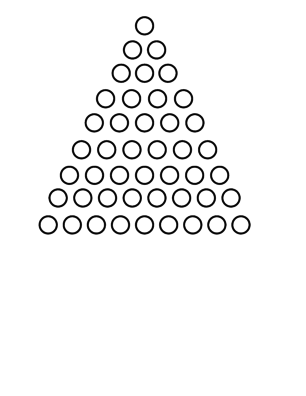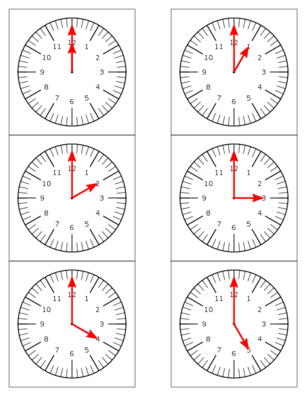# Short Bead StairPrint out of bead stair for coloring

#### Purpose:

To Teach Addition Tables

#### Materials:

• A mat
• A box containing the following bead stair:  -0- 1 red bead -00- 2 green beads -000- 3 pink beads -0000- 4 yellow beads -00000- 5 light blue beads -000000- 6 purple beads -0000000- 7 white beads -00000000- 8 brown beads -000000000- 9 dark blue beads

Each bead bar is strung together with wire. Each number of beads is a set unit. There should be 2 bead stairs for addition exercises.

Teach the addition tables as follows:

 1+1= 9+1= 1+2= 9+2= 1+3= 9+3= 1+4= 9+4= 1+5= 9+5= 1+6= 9+6= 1+7= 9+7= 1+8= 9+8= 1+9= 9+9=

#### Exercise:

The child makes a stair with beads (like in the number rods).  The child chooses any table, such as the 3 table.

1. The child finds 3+1=
2. Places a 3 bar(3 pink beads strung together) and a 1 bar (1 red bead) on a mat
3. Your child counts all of the beads and tells the results: 4 beads altogether
4. He replaces the bar and takes out a 3 bar and a 2 bar (2 green beads strung together).
5. Your child counts the beads and writes down the number 5.
6. You can make a math book with graph paper and write out various addition problems.
7. For beginners make sums less than five.
8. You might need two bead stairs for same number additives, such as 2+2=, 1+1=, 3+3= etc.
Similar Pages:Calendars and Clocks

Become a Patron!

Sign up for our free Newsletter

Privacy policy Your privacy is very important to us and we will never share, sell or lease your e-mail address to a third party for any reason.